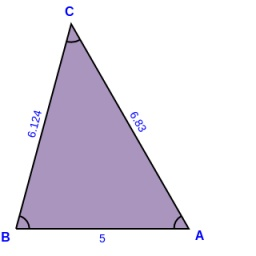# Circumference 6525

The circumference of the triangle is 104 cm. One side is 6 cm longer and 8 cm shorter than the third. Find the lengths of its sides.

a =  34
b =  28
c =  42

### Step-by-step explanation:

a+b+c = 104
a = 6+b
a = c-8

a+b+c = 104
a-b = 6
a-c = -8

Row 2 - Row 1 → Row 2
a+b+c = 104
-2b-c = -98
a-c = -8

Row 3 - Row 1 → Row 3
a+b+c = 104
-2b-c = -98
-b-2c = -112

Row 3 - -1/-2 · Row 2 → Row 3
a+b+c = 104
-2b-c = -98
-1.5c = -63

c = -63/-1.5 = 42
b = -98+c/-2 = -98+42/-2 = 28
a = 104-b-c = 104-28-42 = 34

a = 34
b = 28
c = 42

Our linear equations calculator calculates it.Did you find an error or inaccuracy? Feel free to write us. Thank you!

Tips for related online calculators
Do you have a system of equations and looking for calculator system of linear equations?
Do you want to convert length units?